### Effect of Frequency and Supply Voltage on Iron Losses

Effect of Frequency and Supply Voltage on Iron Losses
The iron losses of a transformer includes two types of losses,
1. Hysteresis loss      and          2. Eddy current loss
For a given volume and thickness of laminations, these losses depend on the operating frequency, maximum flux density and the voltage.
It is known that for a transformer,
V = 4.44 f Φm  N = 4.44 f Bm  A N
Where        A = area
...                Bm   α  (V/f)                                                   .......... For constant A and N
Thus as voltage changes, the maximum flux density changes and both eddy current and hysteresis losses also changes. As voltage increases, the maximum flux density in the core increases and total iron loss increases.
As frequency increases, the flux density in the core decreases but as the iron loss is directly proportional to the frequency hence effect of increased frequency is to increase the iron losses.
Key Point : Thus iron loss increases as the voltage and frequency increases for the transformer.

Example 1 : 1 kv/ 2 KV transformer has 750 W hysteresis losses and 250 W eddy current losses. When the applied voltage is doubled and frequency id halfed, find the new losses.
Solution : The hysteresis loss is given by,
Phα  Bm1.6  f
The eddy current loss is given by,
Peα  Bm2 f2
But         Bmα  (V/f)
...            Phα  (V/f)1.6 x f       and       Peα  (V/f)2 x f2
..           Ph1  /Ph2   = (V1 /V2)1.6 x (f2 /f1)1.6 x (f1/f2)   and    Pe1 /Pe2 = (V1 /V2)2 x (f2 /f1)2 x (f1/f2 )2
Now       V2 = 2 V1   and       f= 0.5 f1
...           750/Ph2   = (0.5)1.6 x (0.5)1.6 x 2   and      250/Pe2  = (0.5)2 x (0.5)2 x (2)2
...            Ph2  = 3446.095 W      and         Pe2 = 1000 W
..Total new iron loss = Ph2  + Pe2  = 4446.095 W

Sponsored links :

#### 11 comments:

1.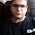thx,you really helped me

1.2.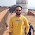transformers are rated in KVA not KW helped me in exam time thank you

3.Really helpful and understanable.. Thanks

4.Thank u very much..it helps me to

understanding rating of T/F.

5.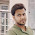Thank you very much.. It was really helpful to me

6.Very clearly explained. Thank u very much!

7.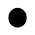If frequency increased with constant voltage - hysteresis loss reduces and eddy current loss unaffected. Please correct

1.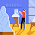But voltage is doubled here dude

8.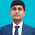iron losses depends on frequency is not correct to say , only hysteresis losses depends on frequency.

9.Tq it is easy to under stand